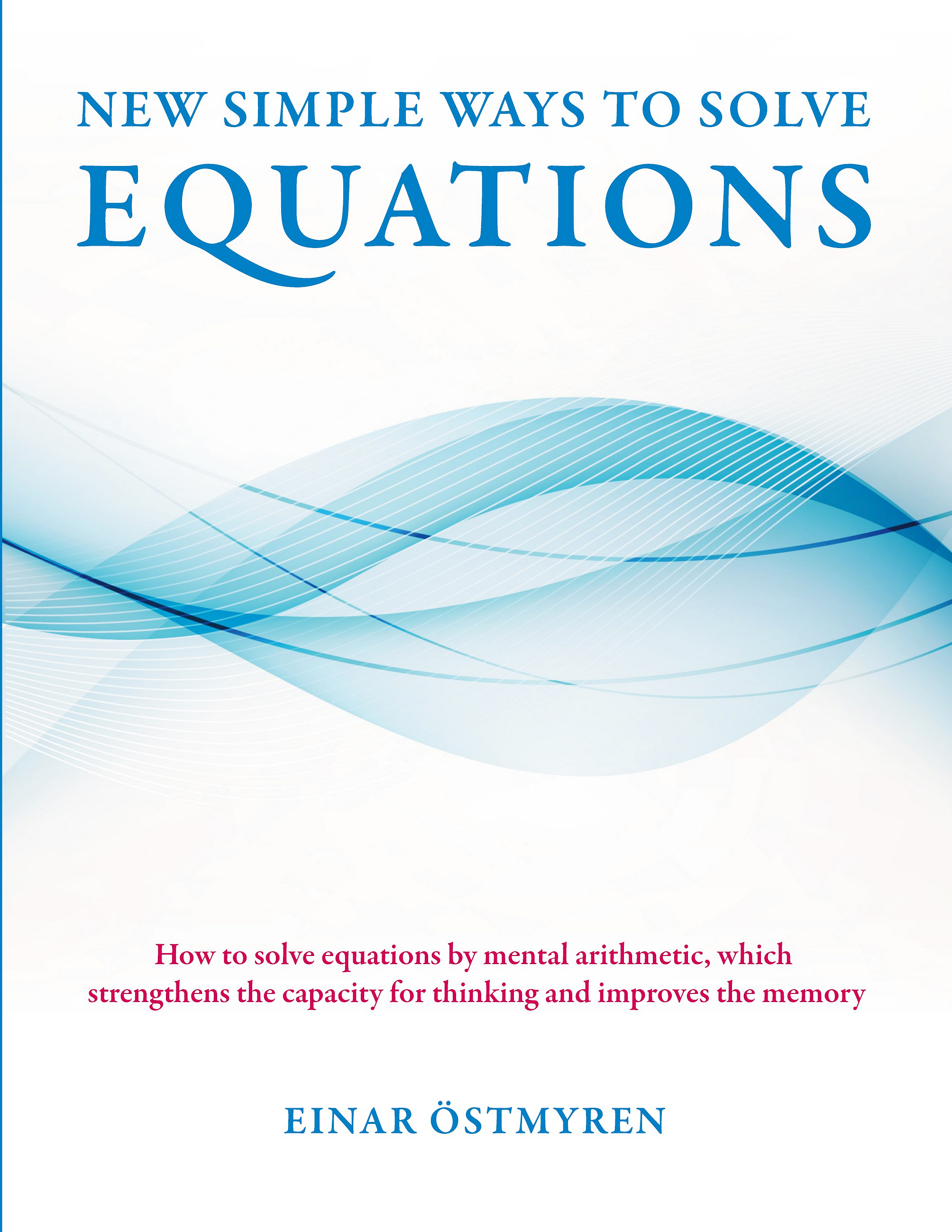The library informs: Välkommen till Hyltebibliotekens e-böcker, med lånekort och en pinkod kan du låna 8 e-böcker varje månad. Mer information hittar du på www.hylte.se/bibliotek .

# Hylte folkbibliotekIsbn: 978-91-7785-287-2
Publisher: Books on Demand
Category:

Accessible since: March 2018

E-book

## New simple ways to solve equations: How to solve equations by mental arithmetic, which strengthens the capacity for thinking and improves the memory

In this book I present a unique formula for quadratic equations, which is a rewriting of the p-q-formula. This rewriting resulted in the equations being solved almost twice as fast by the new formula, when it was compared in a test with the p-q-formula. Another test also showed that the new formula was much faster than the Vedic formula. The new formula is unique because the equations in the test were solved by mere mental calculation, which improves the memory and increases mental agility and intelligence. When I discovered that the middle coefficient in a quadratic equation contains all information about its origin, it led to a rule, that simplified the solving of of all equations. In a quadratic equation the origin could be located, and then it became possible to create a rule how the coefficients were to be split up into factors. By means of this rule and some exercises the answer to an equation can be both calculated and checked regardless of how large the coefficients are.This universal method is intended to be used before the equation is solved by a formula. Since the origin of a quadratic equation could be located, it was also simple to find the origin to other types of equations, and therefore new methods could be created. This led to the fact that a cubic equation could be solved without taking detours like polynomial division, a guess or a test of a root. When the origin of an equation can be located it is as easy to solve a fifth degree equation as a quadratic equation, in the same simple way as unlocking a safe with a key. The purpose of the book is mainly to make it as simple as possible for the students to solve equations, but also to give them a good insight into the origin of an equation.